# Memory Game

Memory Game is a simple random shape matching game that can be created with this Tutorial in Silverlight.

Step 1

Start Microsoft Visual Web Developer 2010 Express, then Select File then New Project... Select "Visual Basic" then "Silverlight Application" from Templates, select a Location if you wish, then enter a name for the Project and then click OK, see below: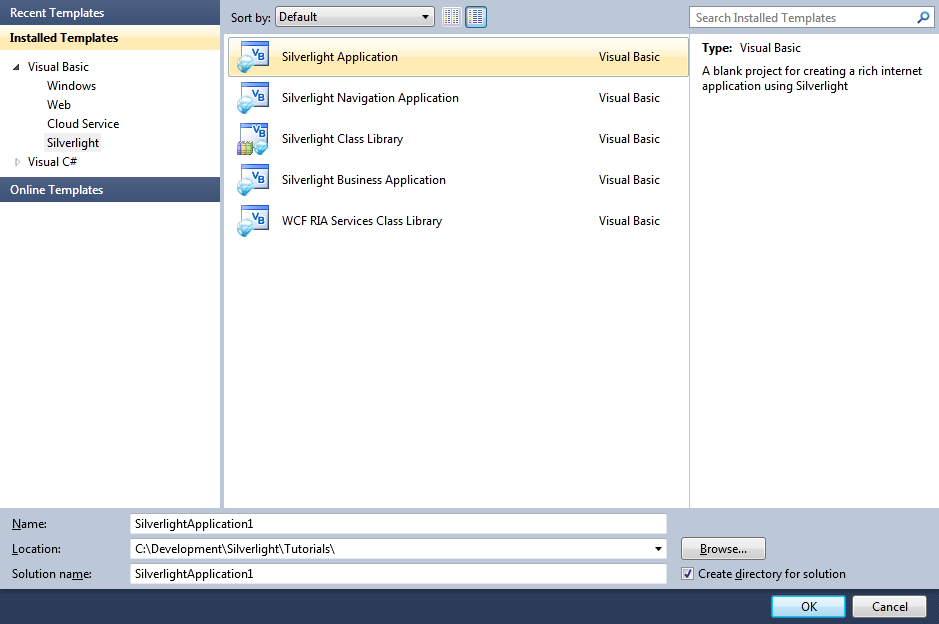Step 2

New Silverlight Application window should appear, uncheck the box "Host the Silverlight Application in a new Web site" and then select the required Silverlight Version, see below: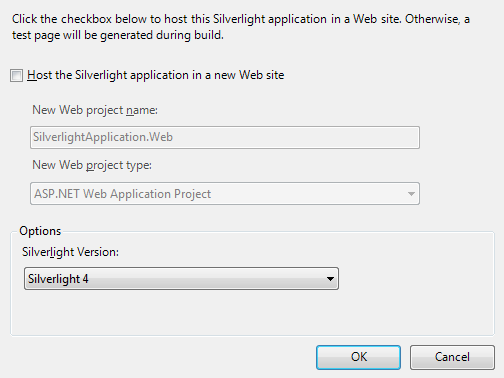Step 3

A Blank Page named MainPage.xaml should then appear, see below:Step 4

Then from the All Silverlight Controls section in the Toolbox select the Canvas control: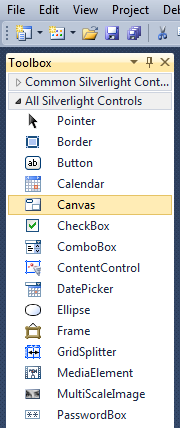Step 5

Draw a Canvas that fill the whole Page or in the XAML Pane between the "<Grid>" and "</Grid>" lines type the following XAML:

```<Canvas Height="300" Width="400" HorizontalAlignment="Left" VerticalAlignment="Top" Name="Page">
</Canvas>
```

See below: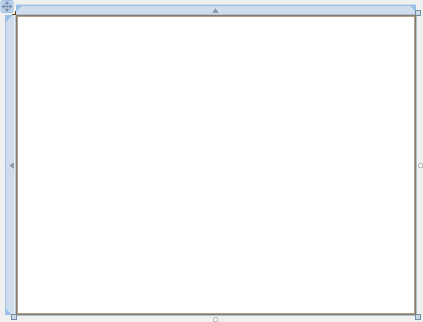Step 6

Then from the Common Silverlight Controls section in the Toolbox select the Grid control: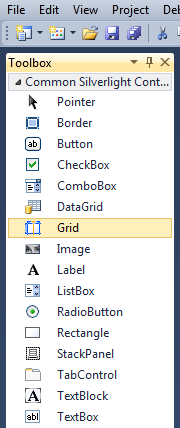Step 7

Draw a Grid on the Canvas by dragging a Grid from the Toolbox onto the Canvas then in the XAML Pane inbetween the "<Canvas>" and "</Canvas>" tags change the "<Grid> line to the following:

```<Grid Canvas.Left="75" Canvas.Top="0" Height="250" Width="250" Name="Display"/>
```

See below:Step 8

Then from the Common Silverlight Controls section in the Toolbox select the Button control: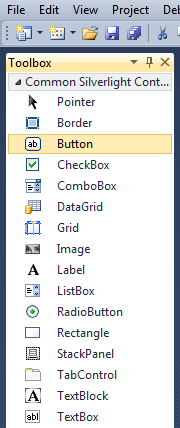Step 9

Draw a Button on the Canvas by dragging the Button from the Toolbox onto the Canvas, then in the XAML Pane below the "<StackPanel>" tag change the "<Button>" line to the following:

```<Button Canvas.Left="163" Canvas.Top="263" Height="23" Width="75" Name="New" Content="New"/>
```

See below:Step 10

Right Click on the Page or the entry for "MainPage.xaml" in Solution Explorer and choose the "View Code" option. In the Code View below the "Inherits UserControl" line type the following:

```Private _moves As Integer = 0
Private _first As Integer = 0
Private _second As Integer = 0
Private _firstButton As Button
Private _secondButton As Button
Private _board(4, 4) As Integer
Private _matches As New List(Of Integer)
```

See Below: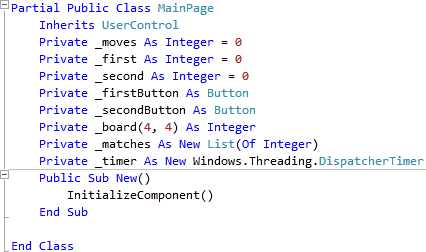Step 11

While still in the Code View for MainPage.xaml, below the "End Sub" for "Public Sub New()" Constructor type the following Function:

```Private Function Shape(ByRef Points As _
System.Windows.Media.PointCollection) As Object
Dim _shape As New Polygon
_shape.Stretch = Stretch.Uniform
_shape.StrokeLineJoin = PenLineJoin.Round
_shape.Points = Points
_shape.Height = 30
_shape.Width = _shape.Height
_shape.Stroke = New SolidColorBrush(Colors.Black)
_shape.StrokeThickness = 4.0
_shape.Margin = New Thickness(5)
Return _shape
End Function
```

See Below: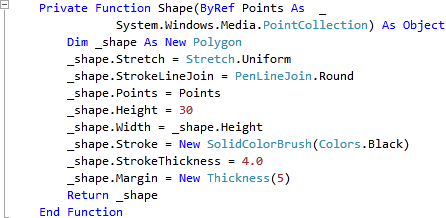Step 12

While still in the Code View for MainPage.xaml, below "End Function" for "Private Function Shape(...)" Function, type the following Function:

```Private Function Card(ByRef Type As Integer) As Object
Dim _points As New System.Windows.Media.PointCollection
Select Case Type
Case 1 ' Circle
Dim _ellipse As New EllipseGeometry
Dim _circle As New Path
_ellipse.Center = New Point(15, 15)
_circle.Data = _ellipse
_circle.Stroke = New SolidColorBrush(Colors.Black)
_circle.StrokeThickness = 4.0
_circle.Margin = New Thickness(5)
Return _circle
Case 2 ' Cross
Dim _lines As New Path
Dim _line1, _line2 As New LineGeometry
Dim _linegroup As New GeometryGroup
_line1.StartPoint = New Point(0, 0)
_line1.EndPoint = New Point(30, 30)
_line2.StartPoint = New Point(30, 0)
_line2.EndPoint = New Point(0, 30)
_lines.Data = _linegroup
_lines.Stroke = New SolidColorBrush(Colors.Black)
_lines.StrokeThickness = 4.0
_lines.Margin = New Thickness(5)
Return _lines
Case 3 ' Triangle
Return Shape(_points)
Case 4 ' Square
Return Shape(_points)
Case 5 ' Pentagon
Return Shape(_points)
Case 6 ' Hexagon
Return Shape(_points)
Case 7 ' Star
Return Shape(_points)
Case 8 ' Rhombus
Return Shape(_points)
Case Else
Return Type
End Select
End Function
```

See Below:Step 13

While still in the Code View for MainPage.xaml, below the "End Function" for "Private Function Card(...)" type the following Subs:

```Private Sub Clear()
If Not _firstButton Is Nothing Then
_firstButton.Content = Nothing
_firstButton = Nothing
End If
If Not _secondButton Is Nothing Then
_secondButton.Content = Nothing
_secondButton = Nothing
End If
_timer.Stop()
End Sub

Private Sub Button_Click(ByVal sender As System.Object, _
ByVal e As System.Windows.RoutedEventArgs)
Dim _btn As New Button
Dim _row, _col, _selected As Integer
If Not _timer.IsEnabled Then
_btn = CType(sender, Button)
_row = _btn.GetValue(Grid.RowProperty)
_col = _btn.GetValue(Grid.ColumnProperty)
_selected = _board(_row, _col)
If _matches.IndexOf(_selected) < 0 Then ' No Match
If _first = 0 Then
_firstButton = _btn
_first = _selected
_firstButton.Content = Card(_selected)
ElseIf _second = 0 Then
_secondButton = _btn
If Not _firstButton.Equals(_secondButton) Then ' Different
_second = _selected
_secondButton.Content = Card(_selected)
If _first = _second Then ' Is Match
MessageBox.Show("Match!", "Memory Game", MessageBoxButton.OK)
If _matches.Count = 16 Then
MessageBox.Show("Well Done! You matched them all in " & _moves & " moves!", _
"Memory Game", MessageBoxButton.OK)
End If
Else ' No Match
_timer.Interval = TimeSpan.FromSeconds(1.5)
_timer.Start()
End If
_moves += 1
_first = 0
_second = 0
End If
End If
End If
End If
End Sub
```

See Below: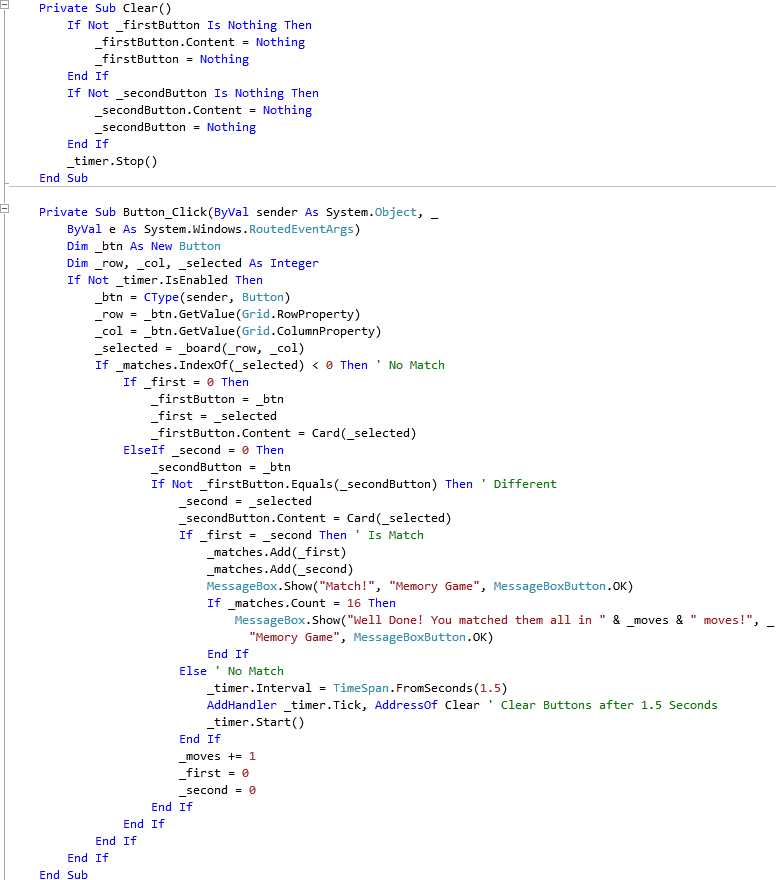Step 14

While still in the Code View for MainPage.xaml, below the "End Sub" for "Private Sub Button_Click(...)" type the following Subs:

```Private Sub Add(ByRef Grid As Grid, ByRef Row As Integer, ByRef Column As Integer)
Dim _btn As New Button
_btn.Content = Nothing
_btn.Margin = New Thickness(5)
_btn.SetValue(Grid.ColumnProperty, Column)
_btn.SetValue(Grid.RowProperty, Row)
End Sub

Private Sub Layout(ByRef Grid As Grid)
Grid.Children.Clear()
Grid.ColumnDefinitions.Clear()
Grid.RowDefinitions.Clear()
For Index As Integer = 0 To 3 ' Setup 4x4 Grid
Next
End Sub
```

See Below: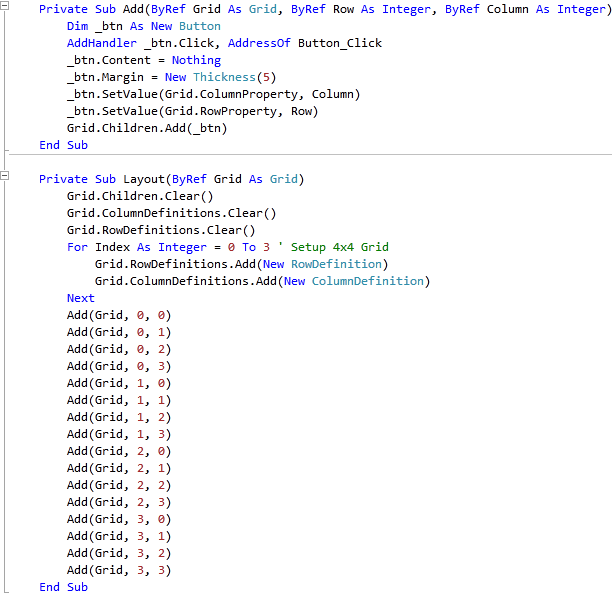Step 15

While still in the Code View for MainPage.xaml, below the "End Sub" for "Private Sub Layout(...)" type the following Function and Sub:

```Private Function Random(ByRef Start As Integer, ByRef Finish As Integer, _
ByRef Total As Integer) As List(Of Integer)
Dim _number As Integer
Dim _numbers As New List(Of Integer)
While _numbers.Count < Total ' Total Numbers
Randomize(Timer) ' Random Number with Seed
_number = Int((Finish * Rnd()) + Start) ' Between Start - Finish
If Not _numbers.Contains(_number) _
Or _numbers.Count < 1 Then ' If Chosen or None
End If
End While
Return _numbers
End Function

Private Sub Choose()
Dim _values, _indices As New List(Of Integer)
Dim _counter As Integer = 0
While _values.Count < 17 ' Get 16 Numbers (2x8)
Dim _numbers As List(Of Integer) = Random(1, 8, 8) ' Random 1 - 8
For _number As Integer = 0 To 7
Next
End While
_indices = Random(1, 16, 16) ' Random 1 - 16
For Column As Integer = 0 To 3 ' Board Columns
For Row As Integer = 0 To 3 ' Board Rows
_board(Column, Row) = _values.Item(_indices(_counter) - 1)
_counter += 1
Next
Next
End Sub
```

See Below: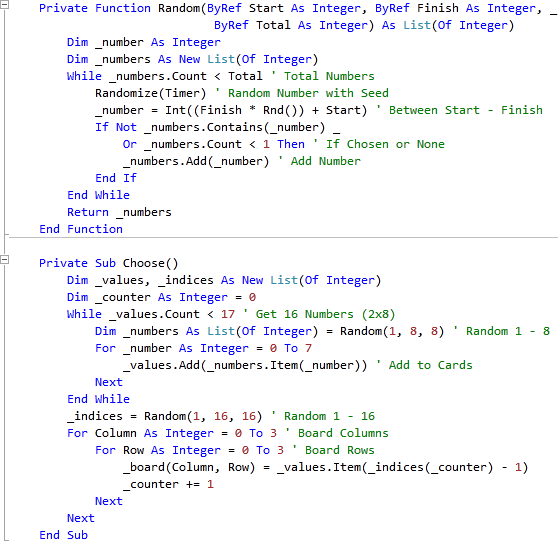Step 16

Return to the Designer View, by selecting the "MainPage.xaml" Tab, or Right Click on the Page or the Entry for "MainPage.xaml" in Solution Explorer and choose the "View Designer" option.
Double Click on the "New" Button Control and type in the New_Click Sub:

```_moves = 0
_matches.Clear()
Layout(Display)
Choose()
```

See Below:Step 17

Save the Project as you have now finished the Silverlight application. Select Debug then Start Debugging or click on Start Debugging:After you do, the following will appear in a new Web Browser window: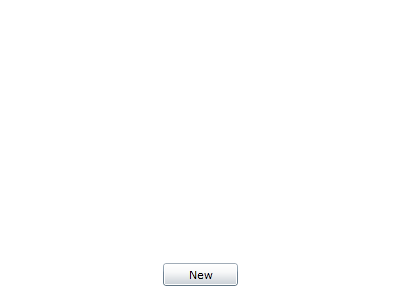Step 18

Click on the New Button and the following will appear:Step 19

Click on any two Buttons to show a Shape, match the shapes to make a pair, do this until all are matched to win, see below: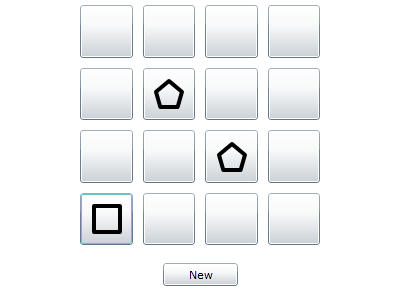Step 20

Close the Browser window by clicking on the Close Button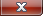on the top right of the Web Browser to Stop the application.

This is a simple game using Shapes for each of the Cards displayed - try changing it to use different shapes, colours or even patterns, you could even make them look more like cards, anything you like!